# Lumped RC Model

#### thisonedude

Joined Apr 20, 2014
52
Hi all. I'm working on the following probelm:

The figure below shows a signal distribution network built in 0.25 μm CMOS whose features are described in Table 4.2 on page 143. All wires are 1.25 μm wide and are routed in metal1. There are loads at each of the lettered nodes in the network that are well-modeled by capacitances of the following values: A,B = 120 fF; C = 180 fF; D,E = 250 fF. For wire resistance, assume wires are made of Aluminum and have a height of 0.36 um.

Draw the equivalent circuit and annotate the values of resistors and capacitors, using lumped R and C models for the network.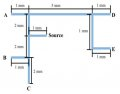Here's table 4.2: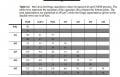I have the following equivalent circuit drawn and also calculated the following resistive values: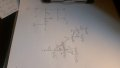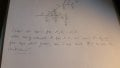So this is the equivalent circuit. does this look like i'm going down the right path? From hear i would use the table and are of the wire to find the capacitances that aren't given to me? and it should be done?

Last edited:

#### thisonedude

Joined Apr 20, 2014
52
Hi all. I'm working on the following probelm:

The figure below shows a signal distribution network built in 0.25 μm CMOS whose features are described in Table 4.2 on page 143. All wires are 1.25 μm wide and are routed in metal1. There are loads at each of the lettered nodes in the network that are well-modeled by capacitances of the following values: A,B = 120 fF; C = 180 fF; D,E = 250 fF. For wire resistance, assume wires are made of Aluminum and have a height of 0.36 um.

Draw the equivalent circuit and annotate the values of resistors and capacitors, using lumped R and C models for the network.

View attachment 95176

Here's table 4.2:

View attachment 95182

I have the following equivalent circuit drawn and also calculated the following resistive values:

View attachment 95193 View attachment 95194

So this is the equivalent circuit. does this look like i'm going down the right path? From hear i would use the table and are of the wire to find the capacitances that aren't given to me? and it should be done?
HI again. So i worked it out and i got the following: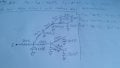the values for the capacitors under numbered nodes were calculated using table 4-2. since we are routing on m1, the top plate is attached to the m1 and the bottom to the substrate so i took the values 30aF/um^2 for the parallel plate cap and 40aF/um for the fringe cap. I then calculated the total cap Cpp+Cfringe for 3 different types of length wire (1mm, 2mm, and 5mm) and got total capacitances of 137.5fF, 275fF, and 687.5fF respectively.

Does it look like i approached this problem properly? Or was i way off as to how i was suppose to solve it and draw the equivalent circuit?

#### namitha

Joined Oct 7, 2016
1
Where you able to find the solution for this? Could you please share the final solution for this?